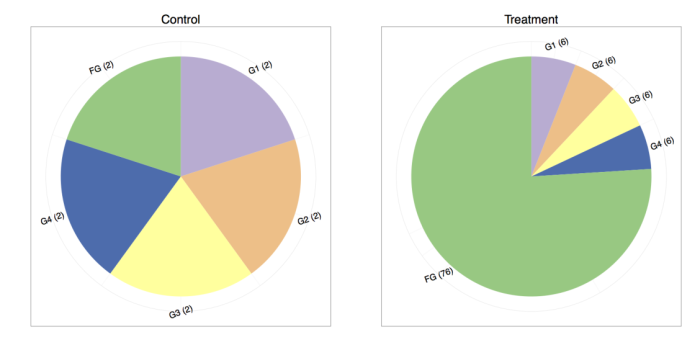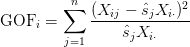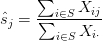# RNA-Seq中样品间的标准化

#### In RNA-Seq, 2 != 2: Between-sample normalization

In this post I will discuss between-sample normalization (BSN), which is often called library size normalization (LSN), though, I don’t particularly like that term. Here, I will refer to it as BSN. The post is guided towards scientists that don’t come from a mathematics background and people who are new to RNA-Seq.

Not so random aside:

After many chats/rants with Nick Bray about RNA-Seq, we decided to abolish the term LSN. The reason being that library size normalization is a pretty misleading phrase, and it is especially confusing to biologists (rightfully so) who think of the library as the cDNA in your sample after prep. LSN term doesn’t address the fact that what you’re measuring is relative and thus counts from different mixtures OR different depths aren’t equivalent.

So, let’s get started. The general point of BSN is to be able to compare expression features (genes, isoforms) ACROSS experiments. For a look at within-sample normalization (comparing different features within a sample), see my previous post. Most BSN methods address two issues:

1. Variable sequencing depth (the total number of reads sequenced) between experiments
2. Finding a “control set” of expression features which should have relatively similar expression patterns across experiments (e.g. genes that are not differentially expressed) to serve as a baseline

# Adjusting for different sequencing depths

Adjusting for total counts is probably the first normalization technique that comes to mind when comparing two sequencing experiments, and indeed, it is inherently used when FPKM is computed without adjusting the “total number of reads” variable in the denominator. The concept is rather simple: I sequenced 1M reads in the first experiment and 2M reads in the second, thus, I should scale my counts by the total number of reads and they should be equivalent. In fact, this is generally what we want to do, but there are a few important caveats.

Consider the (important) case where a feature is differentially expressed. Below we have two toy experiments sequenced at different depths1, each sampling five genes (G1, G2, G3, G4, FG).The control experiment has 10 reads sequenced and the treatment experiment has 100 reads sequenced, so one way to do the total count normalization (which is proportional to FPKM) would be to divide by the total counts. The results are shown in the following table:

GeneControl CountsTreatment CountsControl NormalizedTreatment Normalized
G12.006.000.200.06
G22.006.000.200.06
G32.006.000.200.06
G42.006.000.200.06
FG2.0076.000.200.76

The result is quite preposterous! If one compares the control and treatment proportions, it seems that every gene is differentially expressed. We are typically under the assumption that the majority of the genes do not change in expression. Under that assumption, it is much more plausible that genes one through four remain equally expressed, whereas “funky gene” (FG) is the only differentially expressed gene.

Noting that FG is the only one likely to have changed, our normalization strategy could be different. We might consider normalizing by the sum of the total counts while omitting FG since its funkiness is throwing off our normalization. Thus, for the control sample we would now divide by 8 and in the treatment we would divide by 24:

GeneControl CountsTreatment CountsControl Normalized (-FG)Treatment Normalized (-FG)
G12.006.000.250.25
G22.006.000.250.25
G32.006.000.250.25
G42.006.000.250.25
FG2.0076.000.253.17

And now we are back to a more reasonable looking result where only “funky gene” appears to have changed expression. The astute reader might also note that the relative proportions have changed in the RNA-Seq experiment, but the absolute amount of transcripts from G1-G4 probably did not. Indeed, as an exercise you can scale the “control” experiment to 100 reads and notice that you will still see the effect of all of the genes being differentially expressed if using total count normalization2.

You might be thinking “So.. in this case it was pretty obvious because funky gene is pretty crazy. How do we do this in general?” That’s a great question! Thus, enter the whole slew of RNA-Seq between-sample normalization methods.

# Between-sample normalization techniques

There have been several quite good papers published on between-sample normalization (BSN), some of which I’ve collectedbelow. In almost all the methods, the techniques are simply different ways of finding a “control set” of expression features and using these to estimate your total depth, as opposed to using all of your data to estimate your total depth. While I offered a toy example on why it is important to have a control set of features, more complete examples can be found in Bullard et. al. andRobinson and Oshlack. The basic idea is that highly-variable expression features and differentially expressed features can eat a lot of your sequencing depth, thus throwing off the relative abundance of your expression features.

## Mathematical framework

Let$RNA-Seq中样品间的标准化$ be the observed counts for expression feature$RNA-Seq中样品间的标准化$ (maybe a gene or transcript) in sample$RNA-Seq中样品间的标准化$. We assume$RNA-Seq中样品间的标准化$ is a sample from some distribution with the following property:$RNA-Seq中样品间的标准化$ where$RNA-Seq中样品间的标准化$ is a mean expression rate for feature$RNA-Seq中样品间的标准化$ and$RNA-Seq中样品间的标准化$is the sample specific scaling factor for condition$RNA-Seq中样品间的标准化$. This basically means that every single sample from this experimental condition share some common expression rate, but have a different sequencing depth that scales log-linearly.

Under this model, if we assume$RNA-Seq中样品间的标准化$ is fixed, we can solve for$RNA-Seq中样品间的标准化$ using maximum-likelihood or method-of-moments pretty easily. Unfortunately, as you saw earlier we don’t actually know the value of$RNA-Seq中样品间的标准化$, so we must estimate it.

### PoissonSeq: an example

Instead of summarizing every method, I want to run through a method that I think is simple, intuitive, and under-appreciated:PoissonSeq by Li et. al. We define the set$RNA-Seq中样品间的标准化$ as the set of “control” features that we wish to find. Initially, we set$RNA-Seq中样品间的标准化$ to contain every single feature.

1. Set$RNA-Seq中样品间的标准化$
2. Determine which features are outside of the control set — those with variance relatively large or relatively small. To do this, we compute a goodness-of-fit statistic for every feature:3. Pooling all$RNA-Seq中样品间的标准化$ together, we have a distribution. We can then compute$RNA-Seq中样品间的标准化$ as the “center” of the distribution — basically anything in the inter-quartile range of this distribution.
4. Given$RNA-Seq中样品间的标准化$, estimate$RNA-Seq中样品间的标准化$ by:5. If$RNA-Seq中样品间的标准化$ has converged, you’re done. If not, repeat steps 2 – 4.

The key to this method is the goodness-of-fit statistic. If your goodness-of-fit statistic is large, then you have more variance than you would have expected and thus this particular expression feature shouldn’t be considered as part of your control set.

While this method assumes the Poisson distribution, Li et. al. show that it is still robust under the assumption of the negative binomial (the assumed distribution under most differential expression tools).

## Performance of methods

This is an area that definitely needs work, but is rather difficult to measure performance in (other than simulations, which I have my own gripes with). The size factor is basically a nuisance parameter for differential expression inference and while there has been lots of great work in the area, I’m not familiar with any orthogonally validated “bake-off” papers comparing all existing methods.

That being said, I think it is absolutely negligent to still use total count normalization (or no normalization at all!), as it has been well understood to perform poorly in almost all circumstances.

Among the most popular and well-accepted BSN methods are TMM and DESeq normalization.

## Combining within-sample normalization and between-sample normalization

Every normalization technique that I have seen assumes you are modeling counts, so the assumptions might be violated if you are using them directly on TPM or FPKM. While this is true, I think most techniques will give reasonable results in practice. Another possibility is to apply a BSN technique to the counts, then perform your within-sample normalization. This area has not been studied well, though we are actively working on it.

# List of BSN papers

Here are the most relevant publications that I know of. I probably missed a few. If I missed anything relevant, please post it in the comments. If I agree, I’ll add it :).

## Footnotes

1. I normally hate pie charts, but I think in this case they serve an illustrative purpose. Please use pie charts with caution :).
2. This is where 2 != 2 comes from. You can sequence the same amount of reads, but each read means something different in each experiment.

• 本文由 发表
• 转载请务必保留本文链接：https://www.plob.org/article/9222.html